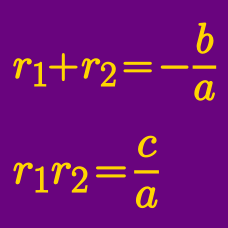Algebra

# Vieta's Formula Problem Solving

Suppose one of the roots of the quadratic equation $x^2+6ax+b=0$ is $$\sqrt{3+\sqrt{8}}.$$ If $$a$$ and $$b$$ are rational numbers, what is the value of $$15ab?$$

What is the sum of the two roots of the following equation: $(3x-1355)^2+4(3x-1355)+5=0?$

$$A$$ and $$B$$ are the two roots of the quadratic equation $$f(x)=0$$. If $$A+B=6$$, what is the sum of the two roots of $$f(2x-21)=0$$?

Let $$a$$ and $$b$$ be the roots of the quadratic polynomial $$x^2-x-5=0.$$ Let $$f(x)$$ be a cubic polynomial such that $$f(a)=a, f(b)=b,$$ $$f(a+b)=a+b,$$ and $$f(ab)=-155.$$ What is the value of $$f(6)$$?

Find the sum of all real solutions to the equation $6 \cdot 4^x - 2057 \cdot 2^x + 3072 = 0.$

×

Problem Loading...

Note Loading...

Set Loading...